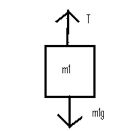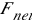# Atwood Machine

The typical setup for the Atwood Machine is below:The string that connects m1 and m2 is considered to be massless and there is no friction in the supporting (top) pulley. Students are asked to solve a problem with a picture like this for either the tension in the string, the acceleration of the system, or both. IF STUDENTS ARE ASKED TO ONLY SOLVE FOR THE ACCELERATION OF THE SYSTEM (BOTH MASSES WILL ACCELERATE AT THE SAME RATE), IT IS SIMPLER TO SOLVE THE PROBLEM USING THE SYSTEM APPROACH. This type of question is common in the multiple choice section of the AP exam.

SYSTEM APPROACH FOR SOLUTION OF ACCELERATION:

Using Newton’s Second Law:

Fnet = ma

a = Fnet/m

Fnet = difference in forces = m1g – m2g

m = sum of masses = m1 + m2

a = (m1g-m2g)/(m1+m2)

Logically, if m2>m1, the acceleration will be downward on the right side and upward on the left side. The converse will also be true: If m1>m2, the acceleration will be upward on the right side and downward on the left side.

Atwood Machine

Atwood Machine More

In the free-response section of the AP exam, students are asked for more than just the acceleration. If students are instructed to solve for the tension, students will have to use the single-body approach:

First, draw the free body diagram for each of the masses:ANDNotice that for each free body diagram, T is present. It is the same tension since it is the same string throughout the system. m1 and m2 are usually different. If they are the same, a = 0, because the forces are balanced.

Approach :

1. Choose a direction of motion. It doesn’t matter which you say goes up or which goes down, but it is logical to choose the larger mass as moving downwards (-a) and the smaller mass as moving upwards (+a). In either case, the magnitude of a is the same.

2. Write an equation using Newton’s 2nd Law for each mass. Each equation will contain T.

3. Solve each equation for T and set equal to each other. This will give you acceleration, a.

4. Substitute back into the equation(s) to give T.

Example:

Find the acceleration of the system and the tension in the string if m1 = 3.0 kg and m2 = 10. kg. Use the diagram from above for m1 and m2. Use 10 m/s2 for g.

1) Since m2 is larger, it will move downward on the right side (-a). m1 will move upward (+a).

2) Fnet = m2or top – bottom = m2a; T – m2g = m2 (-a); T = m2(-a) + m2g; T = -10a + 100= m1or top – bottom = m1a; T – m1g = m1a; T = m1a + m1g; T = 3a + 30

3) -10a + 100 = 3a + 30; 70 = 13a; a = 70/13 = 5.4 m/s2 *

4) T = 3a + 30; T = 3(5.4) + 30 = 46 N * T = -10a + 100; T = -54 + 100 = 46 N *

Try the following problems.

1) Set m1 = 10 kg and m2 = 5 g. Solve for a.

2) Set m1 = 7 kg and m2 = 1 kg. Solve for a.

(source)